# Functions in Python

## Functions in Python with Examples

In this article, I am going to discuss Functions in Python with Examples. Please read our previous article where we discussed Strings in Python with examples. At the end of this article, you will understand the following pointers in detail which are related to python functions.

1. When should we go for function in python?
2. What is a Function?
3. Types of functions in python.
4. How to Create and call a function in Python?
5. Function with and without Parameters
6. Return Keyword in Python.
7. How to Return multiple values from a function in Python?
8. Functions are First Class Objects.
9. Multiple examples to understand the above concepts.
##### General example why the function is required?

Let us understand this with an example. When you go for walk in the early morning, the things you do are

1. Get up from the bed
2. fresh up
3. Tie the shoe
4. Pick the smooth towel
5. Start the walk.

Think of this sequence of steps to do a morning walk. Now when my dad calls for a morning walk, he doesn’t need to explain all these steps each time to me. Whenever dad says, “Get ready for morning walk”, it’s like making a function call. “Morning walk‟ is an abstraction for all the many steps involved.

##### When should we go for function in python?

While writing coding logic, instead of writing like plain text, it’s good to keep those coding statements in one separate block, because whenever required then we can call these. If a group of statements is repeatedly required, then it is not recommended to write these statements each and every time separately.

So, it’s good to define these statements in a separate block. After defining a function we can call it directly if required. This block of statements is called a function. Let us understand more by doing practically.

##### What is a Function?

A function is one that contains a group of statements or a block of code to perform a certain task. The advantages of using functions are:

1. Maintaining the code is an easy way.
2. Code re-usability.

Example: print() is a predefined function in python that prints output on the console

##### Types of functions in python:

There are many categories based on which we can categorize the functions. This categorization is based on who created it.

1. Pre-defined or built-in functions
2. User-defined functions

Predefined or built-in functions: The functions which come installed along with python software are called predefined or built-in functions. We have covered some inbuilt functions in examples of earlier chapters. Some of them are id(), type(), input(), print() etc.

User-defined functions: The functions which are defined by the developer as per the requirement are called user-defined functions. In this chapter, we shall concentrate on these kinds of functions that are user-defined.

##### FUNCTION RELATED TERMINOLOGY
1. def’ keyword – Every function in python should start with the keyword ‘def’. In other words, python can understand the code as part of a function if it contains the ‘def’ keyword only.
2. Name of the function – Every function should be given a name, which can later be used to call it.
3. Parenthesis – After the name ‘()’ parentheses are required
4. Parameters – The parameters, if any, should be included within the parenthesis.
5. Colon symbol ‘:’ should be mandatorily placed immediately after closing the parentheses.
6. Body – All the code that does some operation should go into the body of the function. The body of the function should have an indentation of one level with respect to the line containing the ‘def’ keyword.
7. Return statement – Return statement should be in the body of the function. It’s not mandatory to have a return statement.

Note: After defining a function we can call the function using its name. While calling a function, we need to pass the parameters if it has any as stated in point 4 above.

##### How to Create and call a function in Python?

From creating a function to using it, these are the two things that are done.

1. Defining function
2. Calling function
##### Defining a function in Python

In the function-related terminologies above, it was clearly explained what is what and how are they used. All the points together define the function. To summarize the definition contains – def keyword, name for the function, parentheses, parameters(optional), colon (:), body, return(optional).

###### Syntax to define a function in Python: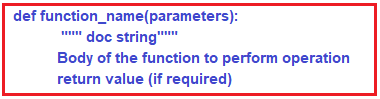###### Example: Define a function that has no parameters (Demo1.py)
```def display():
print("welcome to function")```

Output: No Output

##### Calling a function in Python:

In the above demo1.py example we defined a function with the name ‘display’ with a print statement in it. But when we execute the demo1.py it will not display any output because the function is not called. Hence, function calling is also important along with function definition.

After defining a function, we need to call to execute the function. While calling the function, we have to call with the same name of the function which we used while defining it, otherwise we will get an error.

##### Example: Define a function and call it (Demo2.py)
```def display():
print("welcome to function")

display()
display()
display()```
###### Output: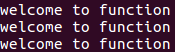Note: When a function is called once then it will execute once, if called twice then it will be executed twice, and so on.

###### Example: Define a function and call it with a different name (Demo3.py)
```def one():
print("welcome to function")
two()```

Output: NameError: name ‘two’ is not defined

##### Function with Parameters in Python:

Based on the parameters, functions can be categorized into two types. They are:

1. Function without parameters
2. Function with parameters
##### Function without Parameters in Python:

A function that has no parameters in the function definition is called a function without parameters. The syntax is given below.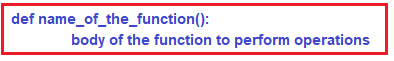###### Example: Function performing addition operation (Demo5.py)
```# defining a function
def sum(a, b):
print("Sum of two values=", (a+b))
# calling function
sum(20,30)```

Output: Sum of two values= 50

###### Example: Check a number is even or odd by using a function (Demo6.py)
```def checking(num):
if num % 2 == 0:
print(num," is even")
else:
print(num," is odd")
checking(12)
checking(31)```
###### Output: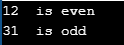##### Return Keyword in Python:

As mentioned above, the return statement is included in the function body, and it returns some results after doing the operations. Some point about the return statement

1. return is a keyword in the python programming language.
2. By using return, we can return the result.
3. It is not mandatory for a function to have a return statement.
4. If there is no return statement in the function body then the function, by default, returns None
###### Syntax to use Return Keyword in Python: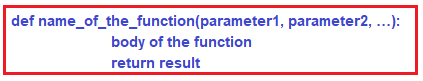###### Example: function returning the value (Demo7.py)
```def sum(a, b):
c = a + b
return c
x=sum(1, 2)
print("Sum of two numbers is: ",x)```

Output: Sum of two numbers is: 3

###### Example: function returning the value (Demo8.py)
```def m1():
print("This function is returning nothing")

# function calling
m1()

x=m1()
print(x)```
###### Output: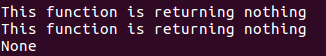##### How to Return multiple values from a function in Python?

In python, a function can return multiple values. If a function is returning multiple values then the same should be handled at the function calling statement as shown in the demo9.py. x and y, two variables are used to capture the values returned by the function m1.

###### Example: Define a function that can return multiple values (Demo9.py)
```def m1(a, b):
c = a+b
d = a-b
return c, d
#calling function
x, y = m1(10, 5)
print("sum of a and b: ", x)
print("subtraction of a and b: ", y)```
###### Output: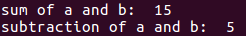##### The function can call another function in Python:

It is also possible in python that a function can call another function. The syntax to call a function from another function is given below.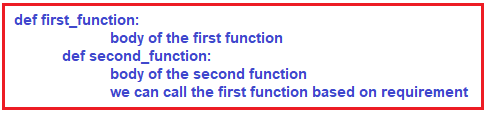###### Example: One function can call another function in python (Demo10.py)
```def m1():
print("first function information")
def m2():
print("second function information")
m1()
m2()```
###### Output: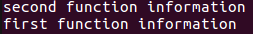In demo10.py, we defined two functions m1 and m2. In function m2, we are calling the m1 function. So finally, we are calling only the m2 function which internally calls the m1 function.

##### FUNCTIONS ARE FIRST CLASS OBJECTS

All functions in Python are first-class functions. To say that functions are first-class in a certain programming language means that they can be passed around and manipulated in the same way as to how you would pass around and manipulate other kinds of objects (like integers or strings). You can assign a function to a variable, pass it as an argument to another function, etc. The distinction is not that individual functions can be first-class or not, but that entire language may treat functions as first-class objects, or may not.

Functions are considered as first-class objects. In python, below things are possible to

1. Assign a function to variables (demo11.py)
2. Pass function as a parameter to another function (demo12.py)
3. Define one function inside another function (demo13.py)
4. The function can return another function (demo14.py)
##### Assigning a function to a variable in Python:

Example: Assign a function to a variable (Demo11.py)

```def add():
print("We assigned function to variable")
#Assign function to variable
#calling function
sum()```

Output: We assigned function to a variable

##### Pass function as a parameter to another function in Python

Example: Pass function as a parameter to another function (Demo12.py)

```def display(x):
print("This is display function")
def message():
print("This is message function")
# calling function
display(message())```
###### Output: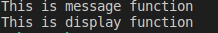##### Define one function inside another function in Python:

Example: function inside another function (Demo13.py)

```def first():
print("This is outer function")
def second():
print("this is inner function")
second()
#calling outer function
first()```
###### Output: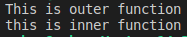Note: If we defined the inner function, then we need to call that inner function in the outer function.

##### The function can return another function in Python:

Example: function can return another function (Demo14.py)

```ef first():
def second():
print("This function is return type to outer function")
return second
x=first()
x()```

Output: This function is return type to outer function

### 2 thoughts on “Functions in Python”

1.Please… Do tutorials on HTML, CSS

2.D is missing in def.

Example: function can return another function (Demo14.py)

ef first():
def second():
print(“This function is return type to outer function”)
return second
x=first()
x()